# PSAT Math : Triangles

## Example Questions

1 2 7 8 9 10 11 12 13 15 Next →

### Example Question #141 : Geometry

Find the height of a triangle if the area of the triangle = 18 and the base = 4.

9

4

1

6

9

Explanation:

The area of a triangle = (1/2)bh where b is base and h is height. 18 = (1/2)4h which gives us 36 = 4h so h =9.

### Example Question #1 : How To Find The Area Of An Acute / Obtuse Triangle

If triangle ABC has vertices (0, 0), (6, 0), and (2, 3) in the xy-plane, what is the area of ABC?

20

12

18

9

10

9

Explanation: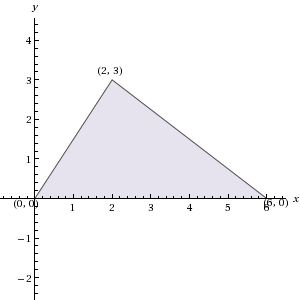Sketching ABC in the xy-plane, as pictured here, we see that it has base 6 and height 3. Since the formula for the area of a triangle is 1/2 * base * height, the area of ABC is 1/2 * 6 * 3 = 9.

### Example Question #64 : Triangles

The height,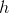, of triangle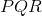in the figure is one-fourth the length of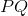. In terms of h, what is the area of triangle?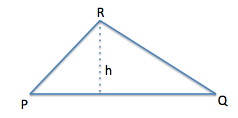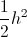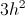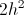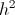Explanation:

If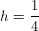*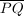, then the length ofmust be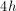.

Using the formula for the area of a triangle (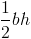), with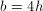, the area of the triangle must be.

1 2 7 8 9 10 11 12 13 15 Next →

### All PSAT Math Resources